### Solution Methods for IVPs: Runge-Kutta Methods

### Runge-Kutta Methods

The Runge-Kutta methods developed by the German mathematicians C. Runge and M.W. Kutta are essentially a generalization of all the previous methods. Consider the following IVP: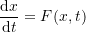Assuming that the value of the dependent variable(say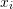) is known at an initial value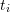, then, the Runge-Kutta methods employ the following general equation to calculate the value ofat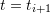, namely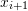with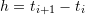: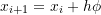where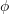is a function of the form: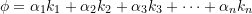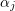are constants while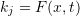evaluated at points within the interval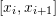and have the form: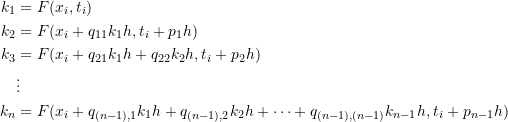The constants, and the forms of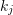are obtained by equating the value ofobtained using the Runge-Kutta equation to a particular form of the Taylor series. The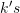are recurrence relationships, meaningappears in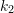, which appears in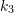, and so forth. This makes the method efficient for computer calculations. The error in a particular form depends on how many terms are used. The general forms of these Runge-Kutta methods could be implicit or explicit. For example, the explicit Euler method is a Runge-Kutta method with only one term with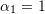and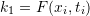. Heun’s method on the other hand is a Runge-Kutta method with the following non-zero terms: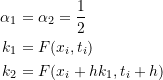Similarly, the midpoint method is a Runge-Kutta method with the following non-zero terms: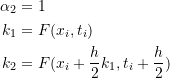The most popular Runge-Kutta method is referred to as the “classical Runge-Kutta method”, or the “fourth-order Runge-Kutta method”. It has the following form: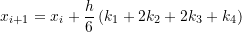with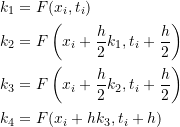The error in the fourth-order Runge-Kutta method is similar to that of the Simpson’s 1/3 rule, with a local error term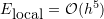which would translate into a global error term of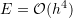. The method is termed fourth-order because the error term is directly proportional to the step size raised to the power of 4.

View Mathematica Code
RK4Method[fp_, x0_, h_, t0_, tmax_] := (n = (tmax - t0)/h + 1;
xtable = Table[0, {i, 1, n}];
xtable[] = x0;
Do[k1 = fp[xtable[[i - 1]], t0 + (i - 2) h];
k2 = fp[xtable[[i - 1]] + h/2*k1, t0 + (i - 1.5) h];
k3 = fp[xtable[[i - 1]] + h/2*k2, t0 + (i - 1.5) h];
k4 = fp[xtable[[i - 1]] + h*k3, t0 + (i - 1) h];
xtable[[i]] = xtable[[i - 1]] + h (k1 + 2 k2 + 2 k3 + k4)/6, {i, 2,
n}];
Data2 = Table[{t0 + (i - 1)*h, xtable[[i]]}, {i, 1, n}];
Data2)


View Python Code
def RK4Method(fp, x0, h, t0, tmax):
n = int((tmax - t0)/h + 1)
xtable = [0 for i in range(n)]
xtable = x0
for i in range(1,n):
k1 = fp(xtable[i - 1], t0 + (i - 1)*h)
k2 = fp(xtable[i - 1] + h/2*k1, t0 + (i - 0.5)*h)
k3 = fp(xtable[i - 1] + h/2*k2, t0 + (i - 0.5)*h)
k4 = fp(xtable[i - 1] + h*k3, t0 + i*h)
xtable[i] = xtable[i - 1] + h*(k1 + 2*k2 + 2*k3 + k4)/6
Data = [[t0 + i*h, xtable[i]] for i in range(n)]
return Data


#### Example

Solve Example 4 above using the classical Runge-Kutta method but with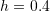.

##### Solution

Recall the differential equation of this problem: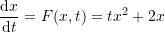Setting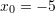,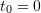,, the following are the values of,,, and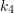required to calculate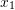: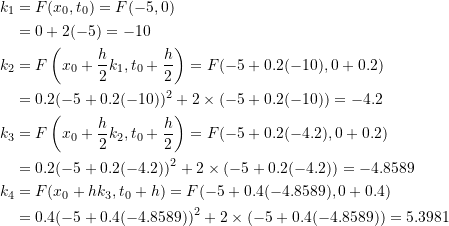Therefore: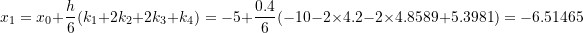Proceeding iteratively gives the values ofup to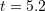. The Microsoft Excel file RK4.xlsx provides the required calculations. The following Microsoft Excel table shows the required calculations: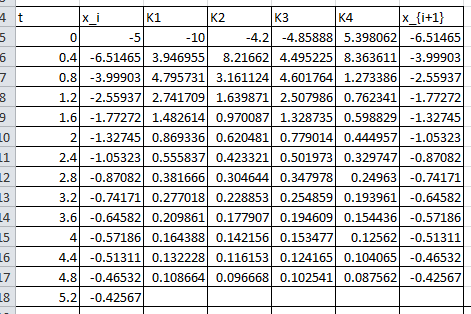The following Mathematica code implements the classical Runge-Kutta method for this problem with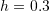. The output curve is shown underneath.

View Mathematica Code
RK4Method[fp_, x0_, h_, t0_, tmax_] := (n = (tmax - t0)/h + 1;
xtable = Table[0, {i, 1, n}];
xtable[] = x0;
Do[k1 = fp[xtable[[i - 1]], t0 + (i - 2) h];
k2 = fp[xtable[[i - 1]] + h/2*k1, t0 + (i - 1.5) h];
k3 = fp[xtable[[i - 1]] + h/2*k2, t0 + (i - 1.5) h];
k4 = fp[xtable[[i - 1]] + h*k3, t0 + (i - 1) h];
xtable[[i]] = xtable[[i - 1]] + h (k1 + 2 k2 + 2 k3 + k4)/6, {i, 2,
n}];
Data2 = Table[{t0 + (i - 1)*h, xtable[[i]]}, {i, 1, n}];
Data2)
Clear[x, xtable]
a = DSolve[{x'[t] == t*x[t]^2 + 2*x[t], x == -5}, x, t];
x = x[t] /. a[]
fp[x_, t_] := t*x^2 + 2*x;
Data2 = RK4Method[fp, -5.0, 0.3, 0, 5];
Plot[x, {t, 0, 5}, Epilog -> {PointSize[Large], Point[Data2]},  AxesOrigin -> {0, 0}, AxesLabel -> {"t", "x"}, PlotRange -> All]
Title = {"t_i", "x_i"};
Data2 = Prepend[Data2, Title];
Data2 // MatrixForm


View Python Code
# UPGRADE: need Sympy 1.2 or later, upgrade by running: "!pip install sympy --upgrade" in a code cell
import numpy as np
import sympy as sp
import matplotlib.pyplot as plt
sp.init_printing(use_latex=True)

def RK4Method(fp, x0, h, t0, tmax):
n = int((tmax - t0)/h + 1)
xtable = [0 for i in range(n)]
xtable = x0
for i in range(1,n):
k1 = fp(xtable[i - 1], t0 + (i - 1)*h)
k2 = fp(xtable[i - 1] + h/2*k1, t0 + (i - 0.5)*h)
k3 = fp(xtable[i - 1] + h/2*k2, t0 + (i - 0.5)*h)
k4 = fp(xtable[i - 1] + h*k3, t0 + i*h)
xtable[i] = xtable[i - 1] + h*(k1 + 2*k2 + 2*k3 + k4)/6
Data = [[t0 + i*h, xtable[i]] for i in range(n)]
return Data

x = sp.Function('x')
t = sp.symbols('t')
sol = sp.dsolve(x(t).diff(t) - t*x(t)**2 - 2*x(t), ics={x(0): -5})
display(sol)
def fp(x, t): return t*x**2 + 2*x
Data = RK4Method(fp, -5.0, 0.3, 0, 5)
x_val = np.arange(0,5,0.01)
plt.plot(x_val, [sol.subs(t, i).rhs for i in x_val])
plt.scatter([point for point in Data],[point for point in Data],c='k')
plt.xlabel("t"); plt.ylabel("x")
plt.grid(); plt.show()
print(["t_i", "x_i"],"\n",np.vstack(Data))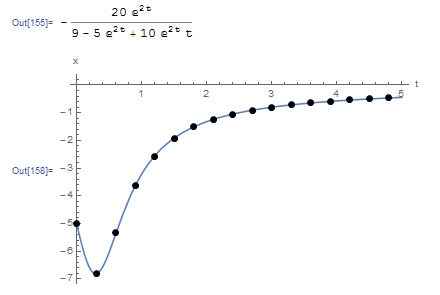The following tool provides a comparison between the explicit Euler method and the classical Runge-Kutta method. The classical Runge-Kutta method gives excellent predictions even when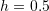, at which point the explicit Euler method fails to predict anything close to the exact equation. The classical Runge-Kutta method also provides better estimates than the midpoint method and Heun’s method above.